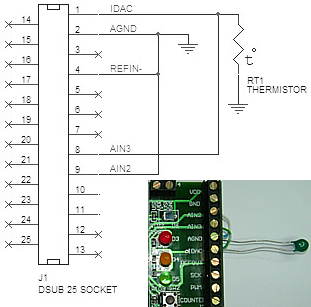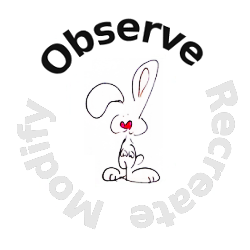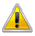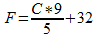The following information is for the EMANT300, EMANT380

# Exercise 7 – Analog Output

## Objective

• Analog Output

## Measure Temperature

A thermistor will be used to measure the forehead temperature. Thermistors are widely used in industrial applications because of their sensitivity, small size, ruggedness and low cost. Thermistors have an electrical resistance that varies non-linearly with temperature. The R-T characteristics of most thermistors can be described by the Steinhart-Hart equation:

1/T = A + B*(Ln R) + C*(Ln R)3

T is the absolute temperature (in Kelvin) and A, B, and C are constants which can be determined by measuring three sets of resistance and temperature values during calibration.

Most thermistors have a negative temperature coefficient (NTC), their resistance decreases with increasing temperature. Thermistors are specified according to its nominal resistance at 25 oC and commonly available thermistors range from 250 ohms to 100 kohms

The thermistor that we are using has the following characteristics

• Nominal resistance @ 25 oC: 10 kohms

• negative temperature coefficient (NTC)

• Steinhart-Hart equation parameters:

• A= 0.001129148

• B= 0.000234125

• C= 8.76741E-8

As the DAQ module Analog Input measures only voltage, we will need to provide a current source to convert the resistance to voltage. The EMANT300 has an 8 bit current DAC (digital to analog converter). As the DAC has 8 bits resolution, we can drive the resistance from 0 to 1mA in 255 steps with increments of about 39uA. In our exercise, we will drive 0.1mA into the thermistor. As the thermistor has a nominal value of 10 kohm at 25 oC, at this temperature the voltage across the thermistor will be (0.1mA * 10 kohm) = 1V.

## Thermistor Connections• Connect the thermistor to the Light Application Adaptor screw terminals labeled AIN3 and AIN2

• Connect a wire from IDAC to AIN3

• Connect a wire from AGND to AIN2

1.Open the Visual Basic solution VB_2010_Solution.sln.

2. Set the Temperature project as the startup project.

3. View the project code by opening the project's Module1.vb

4.If you are using the EMANT380 Bluetooth DAQ, change the parameter to False and COM5 to the COM port you are using, See exercise 1 step 4.

``DAQ.Open(False,"COM5")``

5. Press Ctrl+F5 to Start without Debugging to run the program. Observe the thermistor resistance and temperature value.

6. Use your finger to touch the thermistor. The temperature should change to reflect the higher temperature of your body.

## Program 7.1 Measure Temperature using Thermistor

``````Imports Emant
Module Module1
Sub Main()
Const A As Double = 0.001129148
Const B As Double = 0.000234125
Const C As Double = 0.0000000876741
Dim volt, temp, R As Double
Dim DAQ As Emant300 = New Emant300
DAQ.Open(True,"COM5")
DAQ.WriteAnalog(0.1)
For i = 0 To 9
R = volt / 0.0001
Console.WriteLine(R)
temp = 1 / (A + B * Math.Log(R) + C * Math.Pow(Math.Log(R), 3))
temp = temp - 273
Console.WriteLine(temp)
DAQ.Delay(1000)
Next
DAQ.Close()
End Sub
End Module``````If you are writing this program from scratch, in order to use the Emant300 class, the class library Emant300.dll must be added to the references folder. See exercise 5.

## Constants

Constants are used when you value you have assigned is fixed throughout the program. Unlike Variables, the value cannot be reassigned. Thus it is suitable for A, B and C found in the Steinhart-Hart equation.
Const A As Double = 0.001129148
Const B As Double = 0.000234125
Const C As Double = 0.0000000876741

## Analog Output

``DAQ.WriteAnalog(0.1)``

The parameter 0.1 (variable is double data type) sets the current output to 0.1 mA. Value must be between 0 to 1 mA

## Math.Log Method

``Math.Log(R)``

Returns the natural (base e) logarithm of a specified number. It is one of the methods from the Math Class. The Math Class provides constants and static methods for trigonometric, logarithmic, and other common mathematical functions. The other method we used in this example is

## Math.Pow Method

Returns a specified number raised to the specified power. The following code performs the following calculation (Ln R)3

``Math.Pow(Math.Log(R),3)``

Information on the Math Class can be found at the Microsoft website.

## Measure temperature in oF (optional exercise)Temperature can be measured using either the Centigrade scale or Fahrenheit scale. The Fahrenheit scale is common in the USA whereas most of the other countries adopt the Centigrade or metric scale. If you are developing a temperature measurement solution that will be used in the USA, you will have to show the temperature in oF.

Develop a Visual Basic program that measures the temperature in oF

Hints:

1. Use the earlier temperature sensor program.

2. To convert oC to oF use the following equation